10
3
watching
1,968
views

# An unknown element was tested using a spectroscopic technique. Light was emitted from the sample at a wavelength of 525.6 nm. Calculate the energy of a photon and the energy of one mole of photons of the light.

Step-by-step explanation:

1. The energy of a photon can be calculated using the following equation:

E = hc/λ

where E is the energy of the photon, h is Planck's constant (6.626 x 10^-34 J s), c is the speed of light (2.998 x 10^8 m/s), and λ is the wavelength of the light in meters.

1. To calculate the energy of a photon with a wavelength of 525.6 nm, we first need to convert the wavelength to meters:

λ = 525.6 nm = 525.6 x 10^-9 m

Now we can plug in the values into the equation:

E = hc/λ = (6.626 x 10^-34 J s)(2.998 x 10^8 m/s)/(525.6 x 10^-9 m)

E = 3.771 x 10^-19 J

So the energy of one photon of light with a wavelength of 525.6 nm is 3.771 x 10^-19 J.

To calculate the energy of one mole of photons, we need to multiply the energy of one photon by Avogadro's number (6.022 x 10^23 photons/mol).

E(mol) = E(photon) x N_A

E(mol) = (3.771 x 10^-19 J/photon) x (6.022 x 10^23 photons/mol)

E(mol) = 2.27 x 10^5 J/mol

therefore, the energy of one mole of photons of light with a wavelength of 525.6 nm is 2.27 x 10^5 J/mol.

Step-by-step explanation:
The energy of a photon can be calculated using the following equation:

E = hc/λ

where E is the energy of the photon, h is Planck's constant (6.626 x 10^-34 J s), c is the speed of light (2.998 x 10^8 m/s), and λ is the wavelength of the light.

Substituting the given values, we get:

E = (6.626 x 10^-34 J s)(2.998 x 10^8 m/s)/(525.6 x 10^-9 m)

E = 3.56 x 10^-19 J

Therefore, the energy of a photon with a wavelength of 525.6 nm is 3.56 x 10^-19 J.

To calculate the energy of one mole of photons of the light, we need to use the Avogadro's constant (NA), which is 6.022 x 10^23 particles per mole. The energy of one mole of photons (Emol) can be calculated as follows:

Emol = NA x E

Emol = (6.022 x 10^23)(3.56 x 10^-19 J)

Emol = 2.14 x 10^5 J/mol

Therefore, the energy of one mole of photons of light with a wavelength of 525.6 nm is 2.14 x 10^5 J/mol.

We know that the energy of photon os given by the following equation-

E = hc/λ

Where E = energy

h = Planck's constant= 6.6 × 10^-34 m²kg/s

c = speed of light = 3× 10^8 m/s

λ= wavelength

Energy of one photon = (6.626 x 10^-34 m^2 kg/s x 3 x 10^8 m/s) / 525.6 x 10^-9 m = 3.78 x 10^-19 J

1 mol photons = 6.023 x 10^23 photons

Energy of 1 mol of photons = (6.023 x 10^23 x 6.626 x 10^-34 m^2 kg/s x 3 x 10^8 m/s) / 525.6 x 10^-9 m

= 2.28 x 10^5 J

Hope this one helped you

We know that the energy of photon os given by the following equation-

E = hc/λ

Where  E = energy

h = Planck's constant= 6.6  × 10^-34 m²kg/s

c = speed of light = 3× 10^8 m/s

λ= wavelength

Energy of one photon = (6.626 x 10^-34 m^2 kg/s x 3 x 10^8 m/s) / 525.6 x 10^-9 m = 3.78 x 10^-19 J

1 mol photons = 6.023 x 10^23 photons

Energy of 1 mol of photons = (6.023 x 10^23 x 6.626 x 10^-34 m^2 kg/s x 3 x 10^8 m/s) / 525.6 x 10^-9 m

= 2.28 x 10^5 J

Step-by-step explanation:

Equation for the energy of a photon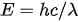E = energy

h = Planck's constant

c = speed of light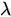= wavelength

Energy of one photon = (6.626 x 10^-34 m^2 kg/s x 3 x 10^8 m/s) / 525.6 x 10^-9 m

3.78 x 10^-19 J

1 mol photons = 6.023 x 10^23 photons

Energy of 1 mol of photons = (6.023 x 10^23 x 6.626 x 10^-34 m^2 kg/s x 3 x 10^8 m/s) / 525.6 x 10^-9 m

2.28 x 10^5 J

Given,

λ(wavelength) = 525.6 nm = 525.6 x 10^-9 m

Part 1 :- calculation of energy of a photon of the light.

We know that,

E = hc/λ -------------> (i)

Here,

E = energy

h = Planck's constant = 6.626 x 10^-34 m^2 kg/s

c = speed of light = 3 x 10^8 m/s

Substituting the above values in equation (i) we have,

E = (6.626 x 10^-34 m^2 kg/s x 3 x 10^8 m/s) / 525.6 x 10^-9 m

=  3.78 x 10^-19 J

Part 2 :- calculation of energy of one mole of photons of the light.

In order to find the energy of one mole of photons of the light we have to multiply the calculated energy from part-1 with avogadro's number (NA).

Therefore, energy of one mole of photons of light =  3.78 x 10^-19 J X NA

= 3.78 x 10^-19 J X 6.022 x 10^23

= 2.28 x 10^5 J

Energy of one photon = 3.78 x 10^-19 J

Energy of 1 mol of photons = 2.28 x 10^5 J

Step-by-step explanation:
Equation for the energy of a photonE = energy

h = Planck's constant

c = speed of light= wavelength

Energy of one photon = (6.626 x 10^-34 m^2 kg/s x 3 x 10^8 m/s) / 525.6 x 10^-9 m

= 3.78 x 10^-19 J

1 mol photons = 6.023 x 10^23 photons

Energy of 1 mol of photons = (6.023 x 10^23 x 6.626 x 10^-34 m^2 kg/s x 3 x 10^8 m/s) / 525.6 x 10^-9 m

= 2.28 x 10^5 JEnergy of a photon=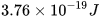Energy of a mole of photon=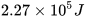Step-by-step explanation:
See the attachmentEnergy of photon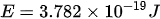Energy of one mole of photon of light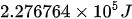Step-by-step explanation:
Given:
h (Planck's constant) =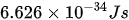c (speed of light) =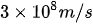(wavelength of light) = 525.6 nm =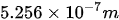Calculating for photon energy: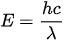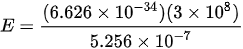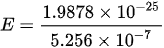Calculating for energy of one mol of photon of light:

We need to multiply the energy of photon by Avogadro's constant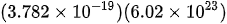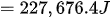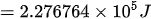Energy of one photon =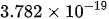J

Energy of one mole photon = 227.749 kJ

Step-by-step explanation:

λ = 525.6nm

c = 3×108m/s

h = 6.626×10−34Js

Energy of one photon is:

E = hc / λ

E = 6.626×10−34 ×3×108 / 525.6×10−9

Energy of one mole photons =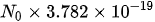=227.749 kJ

Start filling in the gaps now